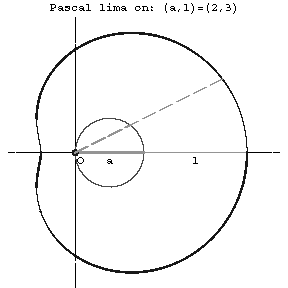# Pascal limaçon

(diff) ← Older revision | Latest revision (diff) | Newer revision → (diff)

A plane algebraic curve of order 4; a conchoid of a circle of diameter $a$ (see Fig.).Figure: p071770a

The equation in rectangular coordinates is

$$(x^2+y^2-ax)^2=l^2(x^2+y^2);$$

in polar coordinates it is

$$\rho=a\cos\phi+l.$$

The coordinate origin is a double point, which is an isolated point for $a<l$, a node for $a>l$, and a cusp for $a=l$ (in this case Pascal's limaçon is a cardioid). The arc length can be expressed by an elliptic integral of the second kind. The area bounded by Pascal's limaçon is

$$S=\frac{\pi a^2}{2}+\pi l^2;$$

for $a>l$ the area of the inner leaf must be counted double in calculating according to this formula. The Pascal limaçon is a special case of a Descartes oval; it is an epitrochoid.

The limaçon is named after Étienne Pascal, who first treated it in the first half of the 17th century.

How to Cite This Entry:
Pascal limaçon. Encyclopedia of Mathematics. URL: http://encyclopediaofmath.org/index.php?title=Pascal_lima%C3%A7on&oldid=42506
This article was adapted from an original article by D.D. Sokolov (originator), which appeared in Encyclopedia of Mathematics - ISBN 1402006098. See original article